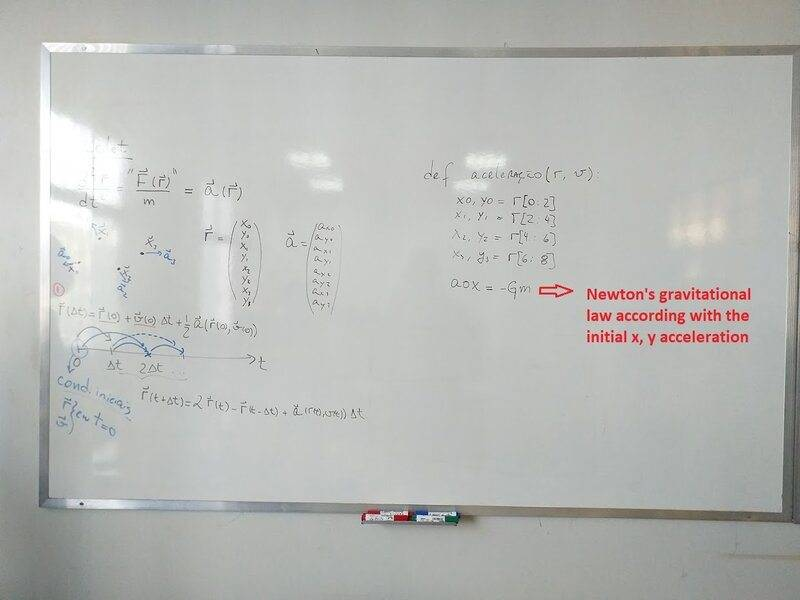# Building a Solar System simulation with python

TheJP78
Homework Statement:
Trying to start.
Relevant Equations:
Basic Verlet (https://en.wikipedia.org/wiki/Verlet_integration#Basic_St%C3%B6rmer%E2%80%93Verlet)

Newton's Gravitational Law
Hey'all. First of all, I'm not fluent in English, so forgive me for the spelling mistakes. So, I'm trying to make a simulation of a solar system using python 3.9. It's not complicated, but my teacher wants me to do it using the Basic Verlet method, and that's what is bugging me. He told me do do divide the code in three steps: Sun-Earth, Earth-Moon, and then the rest of the planets. He also told me the basics to initialize the code:
1. creat a function called acceleration(r, v) that contains the position r(x0, y0, x1, y1, ...) and the velocity v(x0, y0, x1, y1, ...);
2. use the acc(r, v) funtion and the verlet method to calculate the position of the Earth relatively to the sun (the sun will be located in r=v=0);
3. the same case as the above, but now for the earth-moon and the other planets.

He wrote a short instruction for me (linked bellow). My problem is to define the acceleration function. I found a similar code on stack overflow (https://stackoverflow.com/questions...in-python-resulting-in-particles-running-away) and tried to modify it, but didn't work.

If anyone could help me I will be forever grateful.Mentor
Welcome to PF.

tried to modify it, but didn't work.

It would be best if you showed us what you have tried, and describe it in your own words. Posting a whiteboard picture of your instructor's hints helps a little, but we need to see your own work before we can offer tutorial help (that's in the PF rules).

So please post your code that you tried (using code tags) and describe what you think the code should be doing. To use code tags, put [ code ] at the beginining and [ /code ] at the end (but leave out the spaces).

Thanks.

TheJP78
Hi, sorry for the delay. I didn't get much done since my code depends of the acc function. I've spoke to my professor again and he told me how to build the function for the earth-sun system (to introduce the other planets and the moon i will have to add an extra factor G*M*((ri - rj)/mod_(ri-rj)**3) to the accelerations in x and y, and other x, y components do the r vector (x1, y1, x2, y2, etc.)):

Code:
import numpy as np
from math import sqrt

def acc(r):
x0 = r #initial
y0 = r #conditions
mod_r0 = sqrt(x0**2 + y0**2) #module of the r vector
a0x = -G * M_sul * (x0/(mod_r0**3)) #acceleration due to the x component
a0y = -G * M_sul * (y0/(mod_r0**3)) #acceleration due to the y component
acctot = np.array(a0x, a0y) #acceleration vector
return acctot

M_sun is the mass of the sun and G is 6,67408.10^-11 N.kg²/m².

The acceleration will be due to the sun, but when I introduce the moon I will have to consider the force that the moon exerts on the Earth as well.

Now I have to implement the verlet integration method to calculate the orbit of the Earth around the sun and, later on, the other planets orbits and the moon orbit. I think will be something like this:

Code:
r[i] = r + v*dt + a[r]*(dt**2)*0.5 #earth-sun
r[i+1] = 2*r[i] - r[i-1] + a[r]*(dt**2) #other bodies

My professor said the implementation is almost the same as the Euler and Runge-Kutta methods, so I will try to do that way.

Right now, this is all I have done because I have other subjects that are demanding my time. But I will work on it on the weekends to see what more I can do (I have till August 16 to deliver this simulation).

Other thing to add is that I don't need to do an animation of the 8 planets orbiting the sun. It can be just a plot with the eliptics of each one plus the moon orbiting around the earth.

P.S: He also gives me some more instructions, explaining me how to build the function using an example of the pendulum.